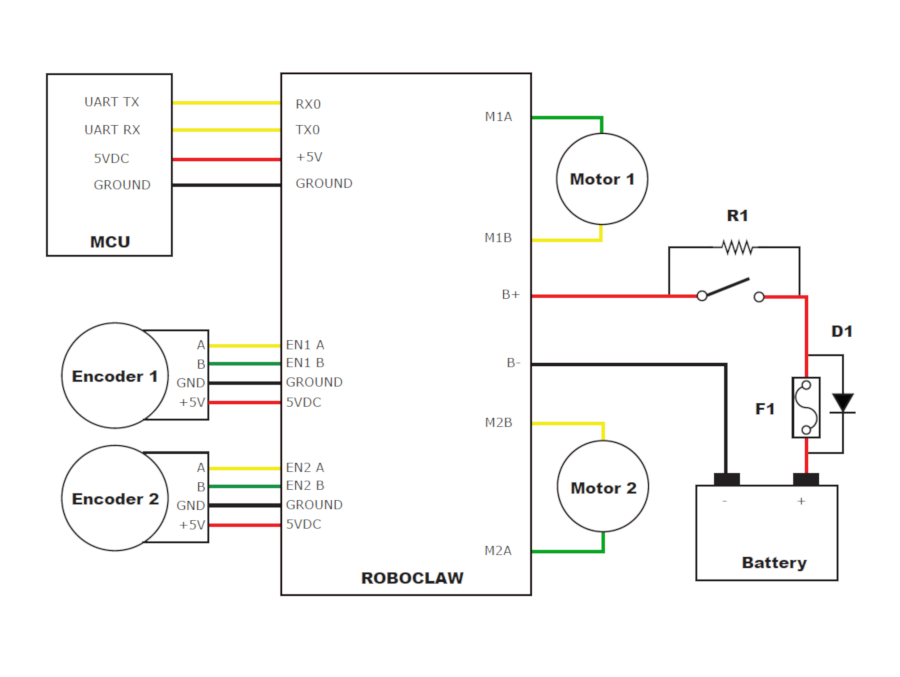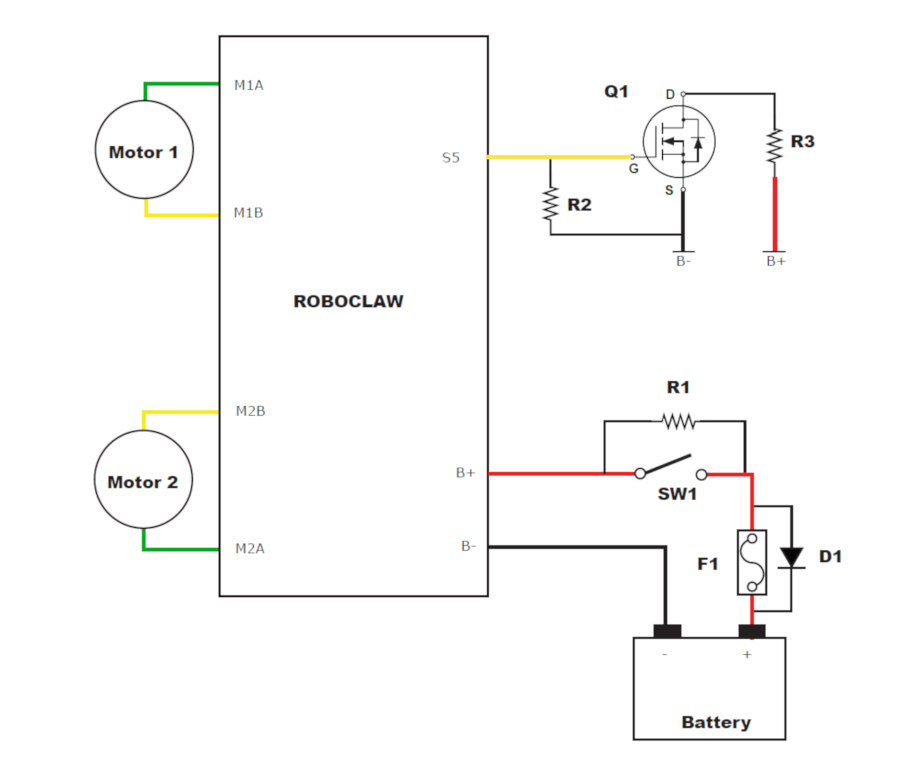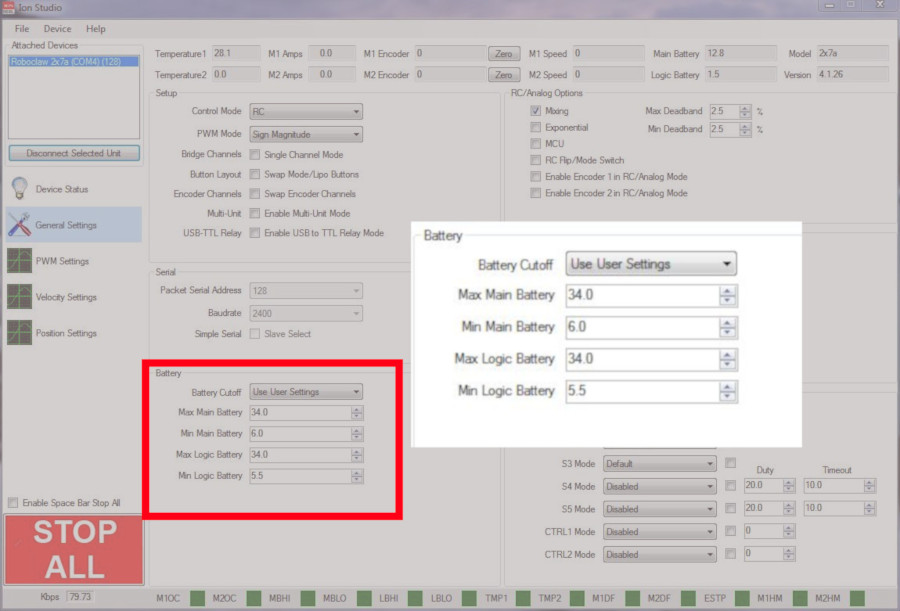# Introduction

When using a RoboClaw of MCP motor controller there are several precautions that must be taken to prevent damage to the controller. Most of them relate to the nature of the regenerative voltage that is generated by motors. This regenerative voltage is generated when motors are slowed down or stopped quickly. The voltage spike comes on suddenly and the voltage can be quite high, high enough to easily damage a motor controller. However, by applying the precautions listed below the motor controller can be easily protected.

# Bypass Diode

One method of preventing voltage spikes from damaging the controller is to use what is referred to as a bypass diode. Under normal operation current flows from the positive side of the power supply to the controller. When a large voltage spike occurs due to regenerative voltage from the motors a bypass diode allows the current to flow in the opposite direction back to the power supply’s positive side. This prevents the high voltage from being presented to the motor controller which would damage it. This protection method only applies when using a battery as a power source, as only a battery will tolerate the reverse current flow. Using this method with a linear or switching power supply is likely to cause damage to the power supply.
To add a bypass diode to a system simply wire an appropriately sized diode in parallel with a switch or fuse on the positive lead of the battery. The cathode side of the diode should be oriented towards the battery and the anode side towards the controller, this will allows the current to flow from the controller to the battery. A power diode rated for 1 to 3 amps of continuous current is recommended for most scenarios. When using a bypass diode it is not necessary to also used a voltage clamping circuit.
In the image shown below the bypass diode is labeled as D1 and is wired in parallel with a fuse labeled F1.
There is one special scenario is which a voltage clamping circuit should be used with a bypass diode. If a system is using a battery that cannot tolerate even the slightest amount of over-charging then a voltage clamp can be used to prevent the regenerative power from over-charging the battery, instead the voltage clamp will dissipate the power as heat in the voltage clamp’s power resistor.Figure 1: The bypass diode in this diagram is labeled D1 and is wired in parallel with the fuse.

## Voltage Clamping

Voltage clamping is another method of shunting large regenerative voltage spikes away from the motor controller. A voltage clamp uses an active element known as a MOSFET. A MOSFET is simply a voltage controlled switch, which in this usage is turned on by a signal from the motor controller. Once the MOSFET is turned on, excess power is sent through a low resistance power resistor which converts the power into heat. This is the protection method that should be used when using a linear or switching power supply with a RoboClaw or MCP controller. The diagram below shows how the circuit is arranged. The current recommend MOSFET is part number BUK965R8 from Digikey. When using a voltage clamping circuit it is not necessary to also use a bypass diode.
In the image shown below the voltage clamp circuit is comprised of the following components: Q1, which is the MOSFET, and two resistors labeled R2 and R3. R3 is the power resistor and R2 keeps the MOSFET turned off when no power is applied to the gate of the MOSFET.Figure 2: The voltage clamp circuit is composed of Q1, R2 and R3 in this diagram.

## Pre-charge Resistor

The RoboClaw and MCP controllers each feature a bank of onboard capacitors. When power is first applied to a system like this the capacitors rapidly charge, which causes a large amount of current to flow in to the controller, this can cause damage. To prevent this from happening a suitably sized resistor is wired in parallel across a switch on the positive lead of the power supply. The resistor allows the board to slowly charge the capacitors before the main power is applied so that a large amount of current does not flow when power is applied to the board.
Note that this configuration of a resistor wired in parallel with a switch will draw power from the power source the entire time power is connected, which is suitable for systems where the power source is disconnected after the system is done being used. For systems with a permanently connected power source a switch can be added in series with the resistor, this allows the pre-charge circuit to be turned on and off. However, in this configuration one must remember to turn the pre-charge switch on first, wait a short period, and then switch on the main power switch.
In the image shown below the pre-charge resistor is labeled R1 and is wired in parallel with a power switch.
It is recommended that you use a pre-charge resistor for system voltages of 24 volts and above.
You can find the value of the resistor needed by diving the desired charing time by 3 and dividing the result by the capacitance of the board’s capacitor bank in farads. Rounding the value up to the nearest standard value of resistor is fine. A 1/4 watt resistor is adequate for the job.
Below is a calculation of the value of a pre-charge resistor for a RoboClaw 2x15A with a 1 second charging time:
Charging time: 1 second

Board capacitance: 400uF, this is .0004 in farads

(charging time / 3) / capacitance in farads

(1 / 3) /.0004 = 825 ohms

The resistor value can be rounded up to 1000 ohms or 1 kohm as this value is commonly available.Figure 3: The pre-charge resistor in this diagram in labeled R1 and is wired in parallel with the switch.

Listed below are the capacitor bank values for all RoboClaw and MCP motor controllers:
 Board Capacitance 2x7A 200uF 2x15A 400uF 2x30A 400uF 2x45A 400uF 2x60A 1080uF 2x120A 2820uF 2x160A 2820uF 2×200 2820uF Solo 30A 400uF Solo 60A 400uF MCP 230x 336uF MCP 26x 400uF MCP 2163 2820uF MCP 2126 2820uF MCP 2166 2820uF MCP 2206 2820uF MCP 2128 2820uF MCP 2168 2820uF

## Maximum Voltage Setting

The final protection that can be put in place is to properly set the maximum voltage for the main battery. When the maximum voltage is exceeded by 1V the motor controller will brake both motor channels. It is also recommended to use a voltage clamp in conjunction with this feature when using a power supply, as the voltage limit does not protect the power supply from regenerative voltage generated from the motors. The maximum main battery voltage can be set in Motion Studio, please see this article to learn about battery settings. The general recommendation is to set the main battery voltage 1 to 2 volts over the maximum voltage of the power source in use.Figure 4: The battery settings panel in Motion Studio.